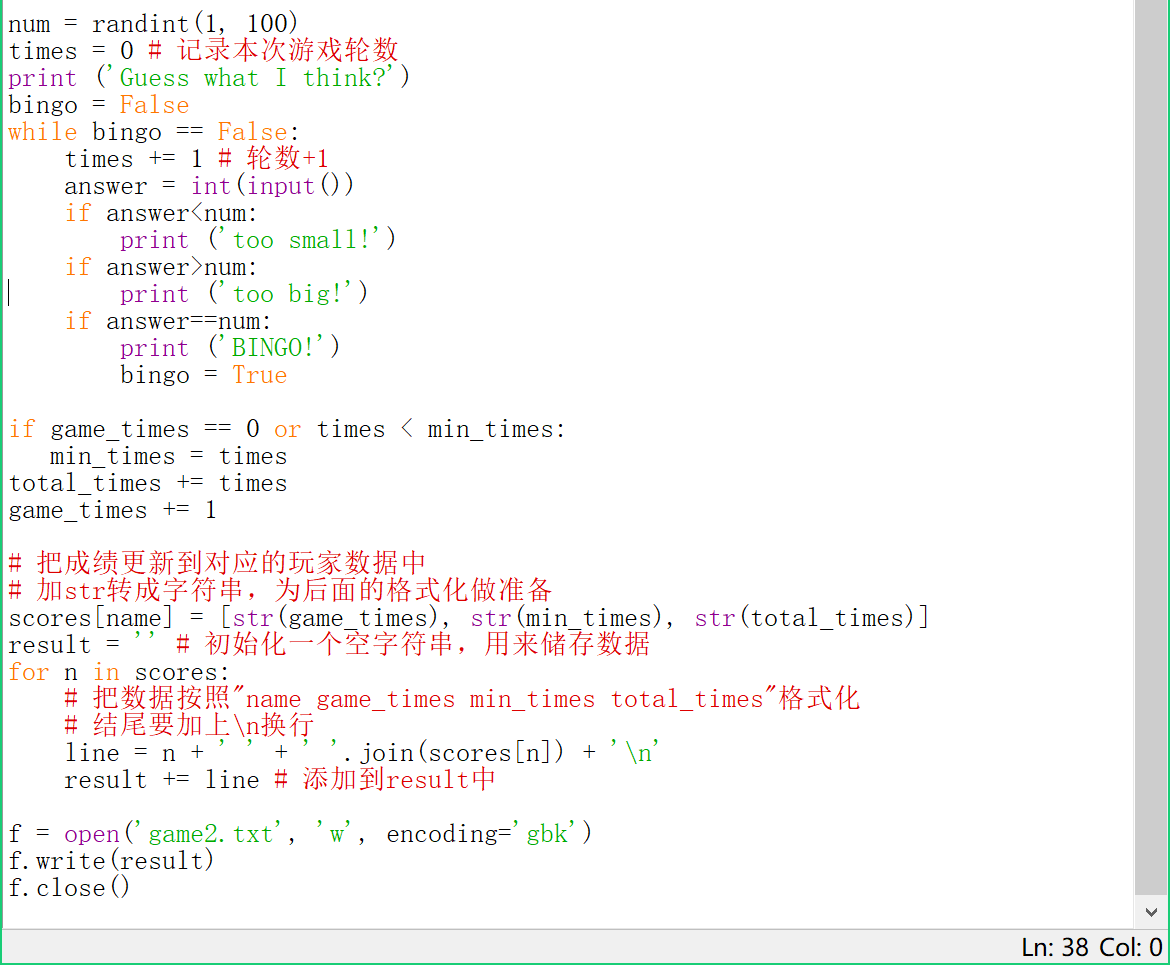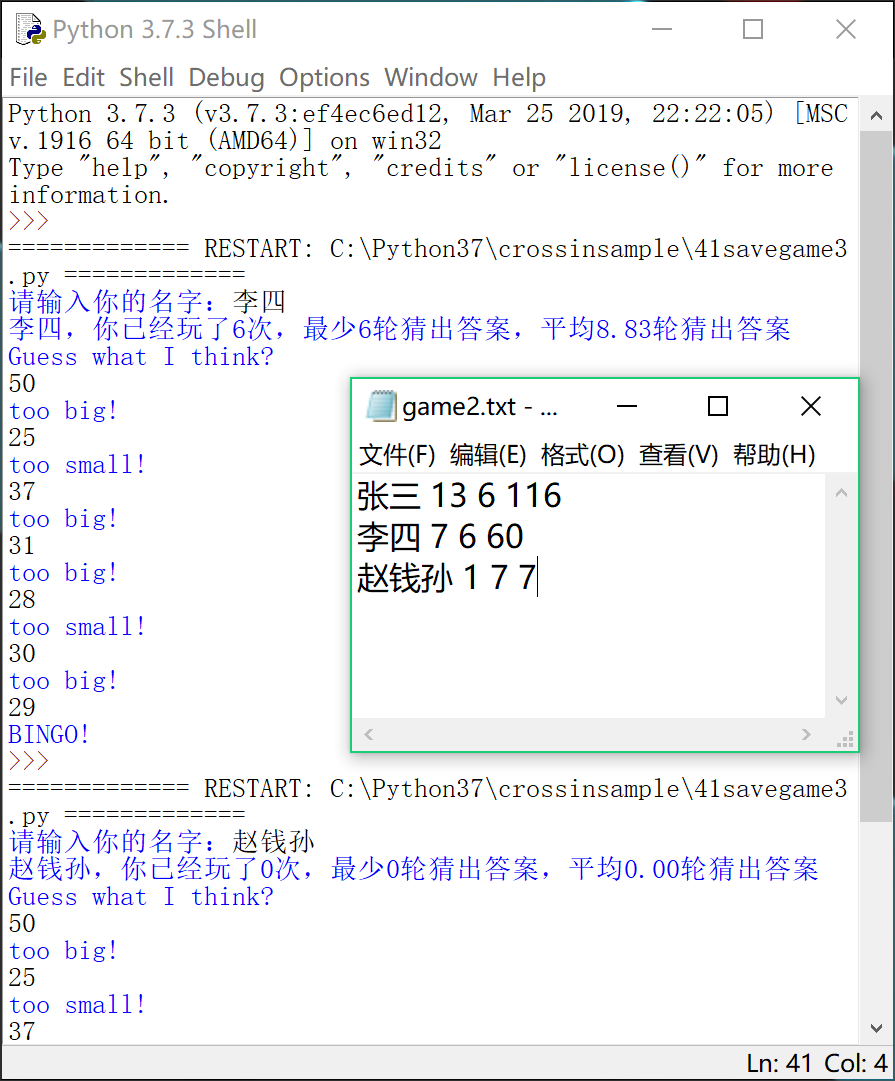## 【Python 第41课】用文件保存游戏（3）

``name = input('请输入你的名字：')``

``lines = f.readlines()``

``````scores = {}
for l in lines:
s = l.split()
scores[s] = s[1:]``````

``score = scores.get(name)``

``````if score is None:
score = [0, 0, 0]``````

``scores[name] = [str(game_times), str(min_times), str(total_times)]``

``````result = ''
for n in scores:
line = n + ' ' + ' '.join(scores[n]) + '\n'
result += line``````